Next: Inverse Z-transform Up: FOURIER AND Z-TRANSFORM Previous: Gaussian examples

## Complex roots

We have seen how a simple two-term filter can destroy the zero frequency or the Nyquist frequency. When we try to destroy any other frequency, we run into a new difficulty--we will see complex-valued signals. Let Z0 take the complex value,where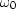is real. Further, choose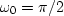and as a result Z0 = i. So the filter (1-Z/Z0)=(1+iZ) has the complex coefficients (1,i), and its output is a complex-valued signal. Naturally this is annoying, because we usually prefer a real output signal.

The way to avoid complex-valued signals is to handle negative frequencythe same way we handle.To do this we use a filter with two roots, one atand one at.The filter (1+iZ)(1-iZ)= 1+Z2 has real-valued time-domain coefficients, namely, (1,0,1). The factor (1+iZ) vanishes when Z=i or,and (1-iZ) vanishes at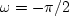.Notice what happens when the filter (1,0,1) is convolved with the time series:the output is zero at all times. This is because bt is a sinusoid at the half-Nyquist frequency,and the filter (1,0,1) has zeros at plus and minus half-Nyquist.

Let us work out the general case for a root anywhere in the complex plane. Let the root Z0 be decomposed into its real and imaginary parts: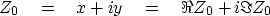(22)
and let the root be written in a polar form: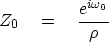(23)
whereand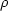are constants that can be derived from the constants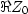andand vice versa. The conjugate root is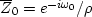.The combined filter is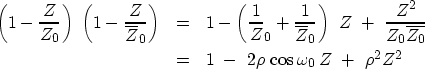(24) (25)
So the convolutional coefficients of this filter are the real values.Taking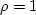, the filter completely destroys energy at frequency.Other values ofnear unity suppress nearby frequencies without completely destroying them.

Recall that to keep the filter response real, any root on the positive-axis must have a twin on the negative-axis. In the figures I show here, the negative axis is not plotted, so we must remember the twin. Figure 10 shows a discrete approximation to the second derivative.ddt2
Figure 10
Approximation to the second difference operator (1,-2,1).

It is like (1-Z)2, but since both its roots are in the same place at Z=1, I pushed them a little distance apart, one going to positive frequencies and one to negative.Next: Inverse Z-transform Up: FOURIER AND Z-TRANSFORM Previous: Gaussian examples
Stanford Exploration Project
10/21/1998